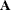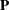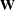### RAM Statement

`RAM` <VAR=variable-list  |  [ variable-list=number-list <, variable-list=number-list …> ],> <ram-entry <, ram-entry …>> ;

where variable-list is a list of variables for the rows and columns of the _A_ and _P_ matrices and the rows of the _W_ vector of the RAM model, number-list is a list of positive integers that denote the order of the specified variables, and ram-entry is a parameter specification for an element in one of the three RAM model matrices. You can specify latent variables in addition to observed variables in the VAR= option.

RAM stands for the reticular action model developed by McArdle (1980). The RAM model implemented in PROC CALIS extends the original RAM model with the specification of the mean vector in the _W_ vector. See the section The RAM Model for details about the model.

The RAM statement specification consists of the list of the variables in the model and the parameters and their locations the RAM model matrices. For example, consider the following simple RAM model specification:

```ram  var= x1-x2 y3,
_A_ 3 1,
_A_ 3 2;
```

In this statement,variables `x1`, `x2`, and `y3` are specified in the VAR= option. The variable order in the VAR= option is important. The same variable order applies to the rows and columns of the _A_ matrix. Next, there are two ram-entries. The first ram-entry specifies that the third variable (`y3`) has a path from the first variable (`x1`). Similarly, the second ram-entry specifies that `y3` has a path from `x2`.

#### Specifying the VAR= Option

In the VAR= option, you specify the list of observed and latent variables in the RAM model. There are two ways to specify the VAR= list. The first way is a simple listing of variables. For example, you specify a total of 18 variables in the RAM model in the following statement:

```ram  var= a b c x1-x5 y1-y10;
```

The order of the variables in this VAR= list is important. The same variable order applies to the rows and columns of the _A_ and _P_ matrices and the rows of the _W_ matrices. Although it is not required to arrange the variables according to whether they are observed or latent in the VAR= list, you can do so for your own convenience. PROC CALIS checks each variable in the VAR= list against the associated data sets to determine whether the variable is observed or latent.

When you specify the parameters in the ram-entries, you represent variables by the variable numbers that refer to the VAR= list. Therefore, it is important to make correct association of the variables and their order on the VAR= list. To this end, you can add some comments in your VAR= list to make the variable numbers explicit. For example,

```ram  var= a      /* 1 */
b      /* 2 */
c      /* 3 */
x1-x5  /* 4-8 */
y1-y10 /* 9-18 */;
```

Another way to specify VAR= list is to provide the variable-lists together with explicit ordering indicated in the number-lists. For example, in the following statement you specify exactly the same variable list as that in the preceding example:

```ram  var= [x1-x5 = 4 to 8, c = 3,  y1-y10 = 9 to 18, a = 1, b = 2];
```

Apart from showing how you can construct the VAR= list in a very general way with the number-lists, there is no particular reason why the variable-lists in the preceding specification are not in either an ascending or a descending order. Perhaps a more natural and useful way to use this type of explicit ordering specification is to place variables in exactly the same order as intended. For example, the following VAR= specification serves as a key of the variable numbers in the subsequent ram-entries:

```ram  var= [x1 = 1, x2 = 2, y1 = 3, y2 = 4, y3 = 5],
_A_   1   2 ,
_P_   2   2 ;
```

With reference to the explicit variable numbers in the VAR= list, you can interpret the `_A_[1,2]` specification immediately as the effect from `x2` to `x1`, and the `_P_[2,2]` specification as the variance of `x2`.

If the VAR= option is not specified in the RAM statement, the n observed variables in the VAR statement are used as the first n variables in the VAR= list. If you specify neither the VAR= option in the RAM statement nor the VAR statement, all n numerical variables in the associated data sets serve as the first n variables in the RAM model matrices. If there are more than n variables used in the ram-entries, the extra variables are all treated as latent variables in the RAM model.

Latent variables generated by PROC CALIS for the RAM model are named in two different ways, depending on whether your RAM model is specified under a MODEL statement. If you do not use the MODEL statement (for example, in situations with single-group analyses), latent variables are named `_Factor1`, `_Factor2`, and so on. If your RAM model is define within the scope of a MODEL statement, latent variables are named `_Mdlk_F1`, `_Mdlk_F2`, and so on, where k is substituted with the model number that is specified in the MODEL statement. For example, `_Mdl2_F1` is a latent factor that is specified under a RAM model within the scope of the MODEL statement with 2 as its model number.

Because data sets might contain nonnumerical variables, implicit variable ordering deduced from the data sets is sometimes obscured. Therefore, it is highly recommended that you use the VAR= option to list all the variables in the RAM model.

#### Specifying a ram-entry

matrix-name | matrix-number row-number column-number <parameter-spec>

A ram-entry is a parameter specification of a matrix element of the RAM model. In each ram-entry, you first specify the matrix by using either the matrix-name or the matrix-number. Then you specify the row-number and the column-number of the element of the matrix. At the end of the ram-entry, you can optionally specify various kinds of parameters in parameter-spec. You can specify as many ram-entries as needed in your RAM model. Ram-entries are separated by commas. For example, consider the following specification:

```ram  var= x1-x2 y3,
_A_ 3 1  1.,
_A_ 3 2;
```

You specify three variables in the VAR= option of the RAM statement. In the first ram-entry, variable `y3` has a path from variable `x1` with a fixed path coefficient 1. In the second ram-entry, variable `y3` has a path from variable `x2`. Because the parameter-spec is blank, the corresponding path coefficient (or the effect from `x2` on `y3`) is a free parameter by default.

##### Specifying the matrix-name or matrix-number

The three model matrices in the RAM model are: _A_, _P_, and _W_. See the section The RAM Model for the mathematical formulation of the RAM model. The matrix-name or matrix-number specifications in the ram-entries refer to these model matrices. You can use the following keywords for matrix-name or matrix-number:

`_A_`, `_RAMA_`, or `1`

for the elements in thematrix, which is for path coefficients or effects

`_P_`, `_RAMP_`, or `2`

for the elements in thematrix, which is for variances and covariances

`_W_`, `_RAMW_`, or `3`

for the elements in thevector, which is for intercepts and means

##### Specifying the row-number and column-number

After you specify the matrix-name or matrix-number in a ram-entry, you need to specify the row-number and column-number that correspond to the intended element of the matrix being specified.

##### Specifying the parameter-spec

You can specify three types of parameters in parameter-spec:

• A free parameter without an initial estimate: blank or parameter-name

You can specify a free parameter for the matrix element in a ram-entry by either omitting the parameter-spec (that is, leaving it blank) or specifying a parameter-name. For example, both of the following ram-entries specify that `_A_[3,1]` is a free parameter in the RAM model:

```_A_  3   1
```

and

```_A_  3   1   beta
```

The difference is that in the latter you name the effect (path coefficient) for the `_A_[3,1]` element as `beta`, while in the former PROC CALIS generates a free parameter name (prefixed with `_Parm` and followed by a unique parameter number) for the specified element. Leaving the parameter-spec blank is handy if you do not need to refer to this parameter in your code. But when you need to specify parameter constraints by referring to parameter names, the parameter-name syntax becomes necessary. For example, the following specification constrains the `_A_[3,1]` and `_A_[3,2]` paths to have equal effects (path coefficients) because they have the same parameter-name `beta`:

```ram  var= x1-x2 y3,
_A_ 3 1  beta,
_A_ 3 2  beta;
```
• A free parameter with an initial estimate: (number) or parameter-name (number)

You can specify a free parameter with an initial estimate in a ram-entry by either specifying the initial estimate within parentheses or specifying a parameter-name followed by the parenthesized initial estimate. For example, both of the following ram-entries specify that `_A_[3,1]` is a free parameter with an initial estimate of 0.3 in the RAM model:

```_A_  3   1   (0.3)
```

and

```_A_  3   1   beta  (0.3)
```

In the latter you name the effect (path coefficient) for the `_A_[3,1]` element as `beta`, while in the former PROC CALIS generates a free parameter name (prefixed with `_Parm` and followed by a unique parameter number). The latter syntax is necessary when you need to specify parameter constraints by referring to the parameter name `beta`. The former syntax is more convenient when you do not need to refer to this parameter in other specifications.

For the latter syntax with a parameter-name specified, you can omit the pair of parentheses or exchange the position of parameter-name and number (or both) without changing the nature of the parameter. That is, you can use the following equivalent specifications for a named free parameter with initial values:

```_A_  3   1   beta  0.3
```

and

```_A_  3   1   .3  beta
```
• A fixed parameter value: number

You can specify a fixed value by simply providing it as the parameter-spec in a ram-entry. For example, in the following syntax you specify that `_A_[3,1]` is a fixed value of 0.3:

```_A_  3   1   0.3
```

The fixed value for `_A_[3,1]` does not change during the estimation. To distinguish this syntax from the initial value specification, notice that you do not put `0.3` inside parentheses, nor do you put a parameter-name before or after the provided value.

#### Notes and Cautions about Specifying ram-entries

• Older versions of PROC CALIS treat a blank parameter-spec in the ram-entry as a fixed constant 1. This is no longer the case in this version of PROC CALIS. Fixed values such as 1.0 must be specified explicitly.

• The row-number and column-number in the ram-entries refer to the VAR= variable list of the RAM statement. An exception is for the _W_ vector, of which the column-number should always be 1 and does not refer to any particular variable.

• When a row-number or column-number in a ram-entry (except for the column-number of _W_) does not have any reference in the VAR= variable list (or is greater than the number of default observed variables when the VAR= option is not specified), PROC CALIS treats the corresponding row or column variable as a latent variable and generates variable names for it.

• The largest row or column number used in any ram-entry should not exceed the sum of observed and latent variables intended in the RAM model. Otherwise, some extraneous latent variables might be created.

#### Default Parameters

It is important to understand the default parameters in the RAM model. First, if you know which parameters are default free parameters, you can make your specification more efficient by omitting the specifications of those parameters that can be set by default. For example, because all exogenous variances and error variances in the RAM model are free parameters by default, you do not need to specify the diagonal elements of the _P_ matrix if they are not constrained in the model. Second, if you know which parameters are default free parameters, you can specify your model accurately. For example, because all the error covariances in the RAM model are fixed zeros by default, you must specify the corresponding off-diagonal elements of the _P_ matrix in the ram-entries. See the section Default Parameters in the RAM Model for details about the default parameters of the RAM model.

#### Modifying a RAM Model from a Reference Model

This section assumes that you use a REFMODEL statement within the scope of a MODEL statement and that the reference model (or base model) is also a RAM model. The reference model is called the old model, and the model that refers to this old model is called the new model. If the new model is not intended to be an exact copy of the old model, you can use the following extended RAM modeling language to make modifications on the model specification. The syntax for modifications is very much the same as the ordinary RAM modeling language (see the section RAM Statement), except that you cannot specify the VAR= option in the RAM statement. The reason is that the VAR= variable list in the new RAM model should be exactly the same as the old model; otherwise, the row-number and column-number in the ram-entries would not have the same references and thus would make model referencing meaningless. Hence, the syntax for respecifying (modifying) the RAM model contains only the ram-entries:

`RAM` ram-entry <, ram-entry …> ;

The syntax of the ram-entry is the same as that of the original RAM statement, with an addition of the missing value specification for the parameter-spec, which denotes the deletion of a parameter location.

The new model is formed by integrating with the old model in the following ways:

Duplication:

If you do not specify in the new model a parameter location (matrix element) that exists in the old model, the old parameter specification is duplicated in the new model.

If you specify in the new model a parameter location (matrix element) that does not exist in the old model, the new parameter specification is added to the new model.

Deletion:

If you specify in the new model a parameter location (matrix element) that also exists in the old model and the new parameter-spec is denoted by the missing value '.', the old parameter specification is not copied into the new model.

Replacement:

If you specify in the new model a parameter location (matrix element) that also exists in the old model and the new parameter is not denoted by the missing value '.', the new parameter specification replaces the old one in the new model.

For example, consider the following two-group analysis:

```proc calis;
group 1 / data=d1;
group 2 / data=d2;
model 1 / group=1;
ram
var = [V1-V6 = 1 to 6, F1 = 7],
_A_ 1 7  1.,
_A_ 7 4  ,
_A_ 7 5  ,
_A_ 7 6  ,
_P_ 1 1  ,
_P_ 2 2  ,
_P_ 3 3  ,
_P_ 7 7  ,
_P_ 4 4  ,
_P_ 5 5  ,
_P_ 6 6  ,
_P_ 1 2  cve12;
model 2 / group=2;
refmodel 1;
ram
_P_ 1 2      .,
_P_ 2 3  cve23;
run;
```

In this example, you specify Model 2 by referring to Model 1 in the REFMODEL statement. Model 2 is the new model which refers to the old model, Model 1. This example illustrates the four types of model integration process by PROC CALIS:

• Duplication: All parameter specifications, except for `_A_[3,7]` and `_P_[1,2]`, in the old model are duplicated in the new model.

• Addition: The new parameter `cve23` is added for the matrix element `_P_[2,3]` in the new model.

• Deletion: The parameter location `_P_[1,2]` and associated parameter `cve12` are not copied into the new model, as indicated by the missing value '.' in the new model specification.

• Replacement: The `_A_[3,7]` path in the new model replaces the same path in the old model with another parameter for the path coefficient. As a results, in the new model paths specified by `_A_[3,7]` and `_A_[2,7]` are constrained to have the same path coefficient parameter `load1`.

PROC CALIS might have generated some default parameters (named with the '_Add' prefix) for the old (reference) model. These default parameters in the old (reference) model do not transfer to the new model. Only after the new model is resolved from the reference model, the REFMODEL statement options, the RENAMEPARM statement, and the model respecification are the default parameters of the new RAM model generated. In this way, the generated parameters in the new model are not constrained to be the same as the corresponding parameters in the old (reference) model. If you want any of these default parameters to be constrained across the models, you must specify them explicitly in the ram-entries of the RAM statement of the reference model so that these specifications are duplicated to the new model via the REFMODEL statement.